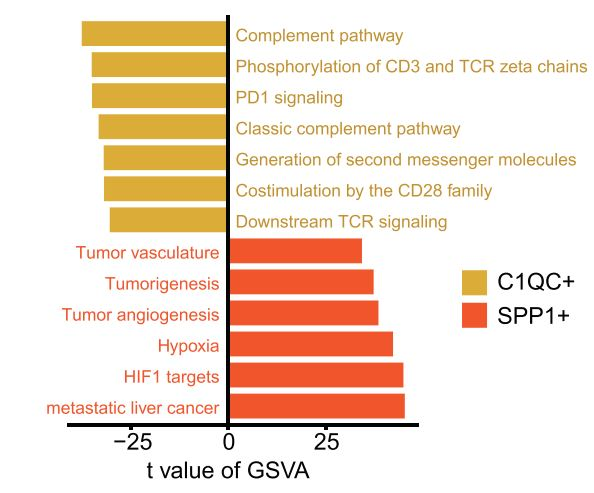# Differentially expressed genes and GSVA pathway enrichment between two cluster of cells

#### Introduction

Whether it is single-cell RNA sequencing or bulk RNA sequencing, we need to perform differential gene expression analysis and GSVA pathway enrichment analysis. The following code is from a single-cell article

#### Code explanation

Load the required library and function. parse_h5ad is used to read anndata from the workflow of Scanpy.As we can see, expression data and meta data are returned.

``````## load data
.libPaths("/data2/csj/tools/Rlib")
library(Seurat)
library(dplyr)
library(monocle)
options(stringsAsFactors=FALSE)
library(reticulate)

require(reticulate)
ad <- import("anndata", convert = FALSE)
}
else{
}
colnames(exp) <- rownames(meta)
return(
list(
expression = exp
)
)
}
``````

Authors calculated log fold change, p value and adjusted p value. "pseudocount.use" was used to avoid zero log. Wilcox.test was used to make test and then p value was adjusted by BH method.

``````############## different expression
## Cluster1 : a vector of Cluster
## Cluster2 : a vector of Cluster

pseudocount.use =1

## log FC
mean_c1 <- as.data.frame(rowMeans(as.matrix(Expression_1)))
colnames(mean_c1) <- "mean_c1"
mean_c2 <- as.data.frame(rowMeans(as.matrix(Expression_2)))
colnames(mean_c2) <- "mean_c2"
log2fc <- data.frame(log2fc = log2(mean_c1\$mean_c1 + pseudocount.use) - log2(mean_c2\$mean_c2 + pseudocount.use))
rownames(log2fc) <- rownames(mean_c1)
log2fc\$gene <- rownames(log2fc)

## wilcox test
group.info <- data.frame(row.names = c(cell_name_1, cell_name_2))
group.info[cell_name_1, "group"] <- "Group1"
group.info[cell_name_2, "group"] <- "Group2"
group.info[, "group"] <- factor(x = group.info[, "group"])
data.use <- h5ad\$expression[, rownames(x = group.info), drop = FALSE]

p_val <- sapply(
X = 1:nrow(x = data.use),
FUN = function(x) {
return(wilcox.test(data.use[x, ] ~ group.info[, "group"])\$p.value)
})

## BH correction

## DE table
return(result)
}
``````

The clusterProfiler package was used to run GSVA analysis.

``````##################################################### GSVA
## Cluster1 : Cluster1
## Cluster2 : Cluster2
## kcdf: kcdf="Gaussian" for continuous and 'Poisson for integer counts'

require(GSVA)
require(GSEABase)
require(clusterProfiler)
library(limma)

## change gene symbol to geneid
gene_entrezid <- bitr(rownames(expression), fromType = "SYMBOL", toType = "ENTREZID", OrgDb = "org.Hs.eg.db")
expression_filt <- expression[gene_entrezid\$SYMBOL,]
rownames(expression_filt) <- gene_entrezid\$ENTREZID
expression_filt <- as.matrix(expression_filt)

rownames(annotation_col) = colnames(res_gsva)

## using limma to conduct DE analysis
f <- factor(annotation_col\$CellType)
design <- model.matrix(~0+f)
colnames(design) <- c("C1","C2")
rownames(design) <- colnames(res_gsva)

fit <- lmFit(res_gsva, design)
cont.matrix=makeContrasts('C1-C2',levels = design)
fit2=contrasts.fit(fit,cont.matrix)
fit2=eBayes(fit2)
gs\$cluster <- ifelse(gs\$t > 0 , "C1", "C2")
return(gs)
}
``````Bar plot showing different pathways enriched in C1QC+ macrophage and SPP1+ macrophage in lung cancer scored per cell by gene set variation analysis
(GSVA). t values are calculated with limma regression

#### Interpretation of results

Although there is not plot function of codes, it is easy to run it in bar plot function.
Figure shows that the SPP1+ TAMs showed preferential expression of genes involved in angiogenesis as a result of validation.

#### References

Cheng S , Li Z , Gao R , et al. A pan-cancer single-cell transcriptional atlas of tumor infiltrating myeloid cells[J]. Cell, 2021, 184(3):792-809.e23.

#### Original

https://github.com/Sijin-ZhangLab/PanMyeloid

#### Donation## 2 thoughts on “Differentially expressed genes and GSVA pathway enrichment between two cluster of cells”

1.yiliu says:

Nice job!

1.user says:

Thanks!